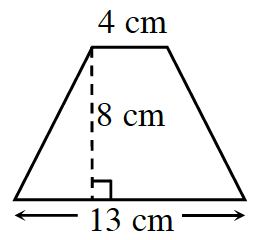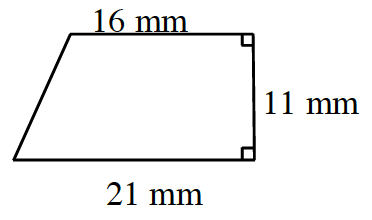### Home > ACC6 > Chapter 6 Unit 6 > Lesson CC1: 6.2.1 > Problem6-71

6-71.

Find the area of each trapezoid below.

1.$\text{The area of a trapezoid is } \\\frac{1}{2}\text{(height)(base 1 + base 2).}$

Here is how Sheila started the problem:

$\frac{1}{2}(8)(13+4)$

Finish her work.

1.See part (a).

$203.5$ square mm